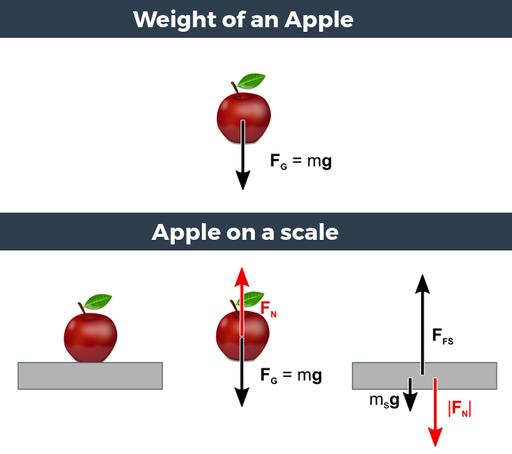Mass is a fundamental property of a physical object, a measure of its inertia (according to Newton's second law of motion). In general, mass is a measure of the amount of matter in an object. The mass of an object is independent of the object's location (the mass of an object is the same on the Earth, the moon or in deep space). The SI unit of mass is kg. The force acting on an object due to gravitation FG=mg is called weight, as illustrated in the upper image of the Figure 1. If the gravitational acceleration and the weight are known, the mass of an object can be determined.

A weighing scale does not measure the weight of an object but rather a force with the same magnitude as the normal force which acts on the object and is supplied by the scale. This situation is depicted in the lower image of Figure 1. When the net force on the apple is zero (apple is not accelerating) the normal force (FN) has the same magnitude as the weight (FG) of the apple. In other words: The scale is pushing upwards with a force equal in magnitude to the weight of the apple. This force (indicated by the red arrow in the free body diagram of the scale) is actually measured by the scale and equals the weight of the apple in case of zero net force.Figure 1: The weight of an apple of mass m is given by FG=mg. Apple on a scale (indicated by the gray rectangle) and the two corresponding free body diagrams (right). FG=mg is the weight of the apple, FN (or N) the normal force, msg the weight of the scale, FFS is the normal force the floor is exerting on the scale.